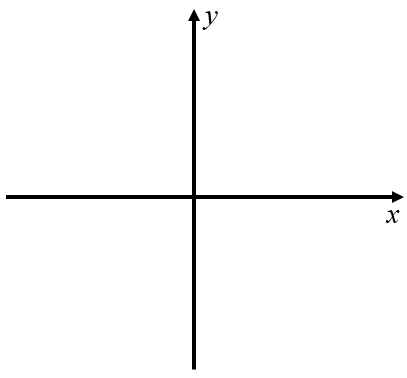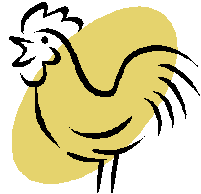# Exam-Style Questions on Proportion

## Problems on Proportion adapted from questions set in previous Mathematics exams.

### 1.

GCSE Higher

At depths below 900 metres, the temperature of the water in the sea is given by the formula:

$$T=\frac{4500}{x}$$

Where $$x$$ is the depth in metres and the temperature of the water is T °C.

(a) A fish swims downwards from a depth of 1000 metres to a depth of 1500 metres. How many degrees colder is the sea water at 1500m than 1000m?(b) Roughly sketch the shape of a graph that shows that T is inversely proportional to $$x$$.

### 2.

GCSE Higher

The Billing triplets are planting seedlings on the first day of the month. The three of them take two hours to plant 300 seedlings.(a) On the second day of the month the triplets are joined by their friend Billy who helps them. Working at the same rate, how many plants should the four of them be able to plant in two hours?

(b) Working at the same rate, how much longer would it take four people to plant 1000 seedlings than it would take five people?

(c) Billy says that it took two hours for three people to plant 300 seedlings. If I assume they work all day, then in one day three people will plant 3600 seedlings because 300 × 12 = 3600.

Why is Billy's assumption not reasonable? What effect has Billy's assumption had on his answer?

### 3.

GCSE Higher

A Primary school asked 9 children to remove the weeds from the school gardens. The gardens measured 100m2 in total and the job was completed in 10 hours (spread over many weeks).

(a) The school was asked to find volunteers to weed another garden measuring 250m2 at a nursing home. This work has to be completed in 15 hours.

Calculate the least number of children the school needs to find for this work.

(b) State one assumption you have made in your answer to part (a). How would your answer to part (a) change if you did not make this assumption?### 4.

GCSE Higher

People are paid to paint polygons.

There are eight people in the polygon painting posse.

All of the people paint at the same rate.

When all of the people are painting, they can paint all of the polygons in the palace in ten days.

The table shows the number of people painting each day:

 Day 1 Day 2 Day 4 All other days Number of people painting 3 6 7 8

Work out the total number of days taken to paint all of the polygons in the palace.

### 5.

GCSE Higher

The following table shows corresponding values for two variables $$x$$ and $$y$$.

 x 1 2 3 4 y 5 $$1 \frac14$$ $$\frac{5}{9}$$ $$\frac{5}{16}$$

(a) If $$y$$ is inversely proportional to the square of $$x$$ find an equation for $$y$$ in terms of $$x$$.

(b) Find the positive value for $$x$$ when $$y = 20$$.

### 6.

GCSE Higher

9 cup cakes weigh a total of 204 g.

There are 420 calories in 100 g of cup cakes.

How many calories are there in one cup cake?### 7.

GCSE Higher

(a) Sketch a graph on the axes below left that shows that $$y$$ is directly proportional to $$x$$.(b) Sketch the graph of $$y = -x^3$$ on the axes above right.

(c) It is possible to draw many rectangles that have area $$48 cm^2$$.

Draw a graph to show the relationship between length and width for rectangles with area $$48 cm^2$$ and sides less than $$50cm$$.

### 8.

GCSE Higher

At a constant temperature, the volume of a gas $$V$$ is inversely proportional to its pressure $$p$$. By what percentage will the pressure of a gas change if its volume increases by 15% ?

### 9.

GCSE Higher

Which of the following statements are correct if $$xy = c$$ and $$c$$ is a constant.

• (a) $$y$$ is directly proportional to $$x$$
• (b) $$y$$ is directly proportional to $$\frac{1}{x}$$
• (c) $$y$$ is inversely proportional to $$\frac{1}{x}$$
• (d) $$x$$ is directly proportional to $$y$$

### 10.

GCSE Higher

Henrietta sells the eggs that the chickens on her farm lay.

She has 220 chickens and each chicken lays 5 eggs each week.

Henrietta gives each chicken 80 grams of chicken feed each day which costs £5.50 for a 20 kg bag.

Work out the cost of the chicken feed for every box of six eggs she sells.### 11.

GCSE Higher

If $$a$$ is inversely proportional to $$b$$ and $$b$$ is directly proportional to $$c^2$$ find a formula for $$a$$ in terms of $$c$$ given that $$a=20$$ and $$c = 4$$ when $$b = 8$$.

If you would like space on the right of the question to write out the solution try this Thinning Feature. It will collapse the text into the left half of your screen but large diagrams will remain unchanged.

The exam-style questions appearing on this site are based on those set in previous examinations (or sample assessment papers for future examinations) by the major examination boards. The wording, diagrams and figures used in these questions have been changed from the originals so that students can have fresh, relevant problem solving practice even if they have previously worked through the related exam paper.

The solutions to the questions on this website are only available to those who have a Transum Subscription.

Exam-Style Questions Main Page

Search for exam-style questions containing a particular word or phrase:

To search the entire Transum website use the search box in the grey area below.